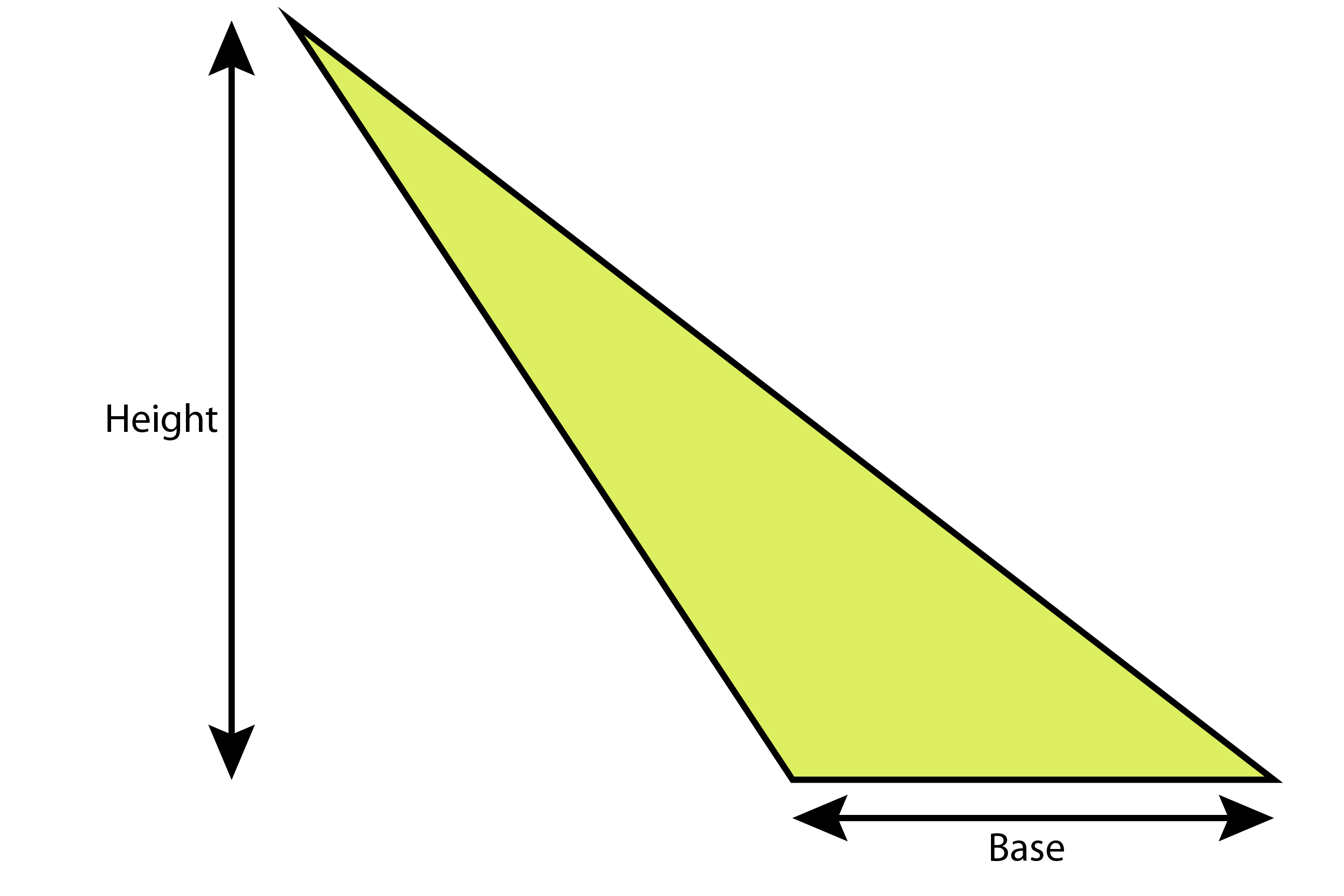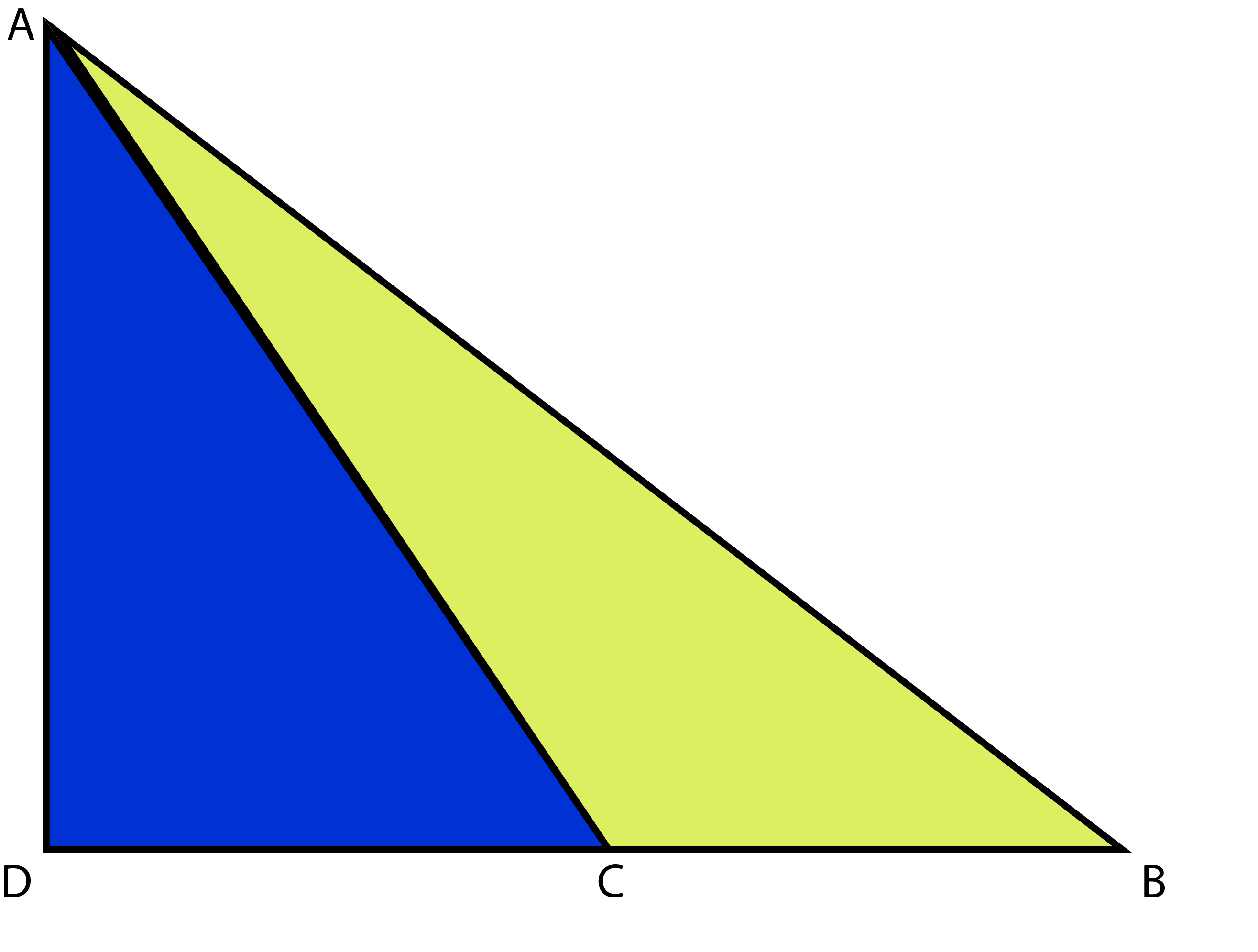# Slanting triangles

Even for triangles shaped as below, the area of a triangle = base x height and half it.The proof (but you don’t need it) is as follows:

Redraw the above diagramArea of triangle ABC=Area triangle ADB-Area triangle ADC

Area of triangle ABC=1/2timesDBtimesAD-1/2timesDCtimesAD

You can remove 1/2AD

Area of triangle ABC=1/2AD(DB-DC)

And because DB-DC=CB

We can now say:

Area of triangle ABC=1/2ADtimesCB

NOTE:

Slanting triangles are rare and it’s still best to think of areas of triangles in a rectangle.